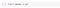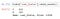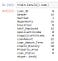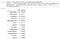# Python Pandas Cheat Sheet

Every day use of pandas functions as a data scientist

Pandas is a python library used in data manipulation ( create, delete, and update the data).

It is one of the most commonly used libraries for data analysis in python. Pandas offer data structures and operations for manipulating numerical and time-series data.

Pandas First Steps

Pandas is an easy package to install. Open up your terminal program (for Mac users) or command line (for PC users) and install it using either of the following commands:

`conda install pandas`

OR

`pip install pandas`

Alternatively, if you’re currently viewing this article in a Jupyter notebook you can run this cell:

The `!` at the beginning runs cells as if they were in a terminal.

To import pandas we usually import it with a shorter name since it’s used so much:importing pandas into jupyer and ‘pd’ stands alias name for pandas.

For this excersis taken dataset of Loan Prediction and can download the dataset from : https://datahack.analyticsvidhya.com/contest/practice-problem-loan-prediction-iii/#ProblemStatement

1. Import necessary Libraries

2. Load dataset (Test & Train)

# Read train and test dataset

a) The first thing to do when opening a new dataset is print out a few rows to keep as a visual reference. We accomplish this with `.head()`:

b) `.head()` outputs the first five rows of your DataFrame by default, including column header and the content of each row.

c) But we could also pass a number as well: `.head(3)` would output the top 3 rows.

4. tail()

`.tail()` outputs the last five rows of your DataFrame by default, including column header and the content of each row.

But we can also pass a number as well: `.tail(2)` would output the top 2 rows.

5. shape

Gives the size of the data frame in the format (row, column).

6. Info()

prints the column header and the data type stored in each column. It also gives the number of non-null values and the memory the data takes.

7. dtypes()

Pandas` DataFrame.dtypes` attribute to find out the data type (dtype) of each column in the given dataframe.

8. Count()

Pandas` dataframe.count()` is used to count the no. of non-NA/null observations across the given axis. It works with non-floating type data as well.

9. Value_counts()

Pandas` .value_counts()` function returns object containing counts of unique values. The resulting object will be in descending order so that the first element is the most frequently-occurring element. Excludes NA values by default.Out of 614 data points, Y repeating 422 times & N 192 times

10. Unique()

a) shows all the non-repeating values of a particular column.

b) Pandas` unique()` function return unique values in the feature. Uniques are returned in order of appearance, this does NOT sort.

11. Printing Column Names

To get all the column headers of a Pandas DataFrame as a list, `df.columns.values` attribute will return a list of column headers.

12. Describe()

Pandas `describe()` is used to view some basic statistical details like mean, median, standard deviation, and percentiles of all the numerical values in your dataset.

13. Missing Values()

In Pandas missing data is represented by two value:

• None: None is a Python singleton object that is often used for missing data in Python code.
• NaN : NaN (an acronym for Not a Number), is a special floating-point value recognized by all systems that use the standard IEEE floating-point representation

In order to check missing values in Pandas DataFrame, we use a function `isnull()` and `notnull()`. Both function help in checking whether a value is `NaN` or not. These function can also be used in Pandas Series in order to find null values in a series.No of Missing values in each featureNo of Missing values in each feature and corresponding percentage. By using missing value percentage can take decision wheather to drop feature or not.